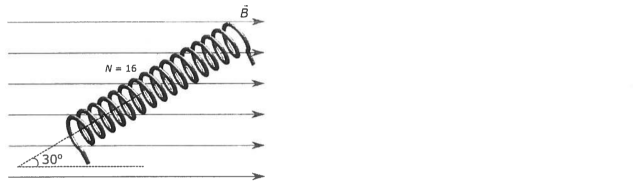Problem: A solenoid with N = 16 turns, length L = 0.30 m, and cross-sectional area  A = 2.0 x 10 -3 m2 sits at an angle Φ = 30° with respect to a uniform magnetic field of magnitude  B = 2.0 x 10-4 T, as shown in the figure. The solenoid carries a current  I and experiences a torque of magnitude 6.4 x 10-9 N•m. What is the magnitude of I? (a) 1.2 mA (b) 0.6 mA (c) 32 mA (d) 2.0 mA (e) 0.5 A (f) none of the above

FREE Expert Solution
81% (434 ratings)
Problem Details

A solenoid with N = 16 turns, length L = 0.30 m, and cross-sectional area  A = 2.0 x 10 -3 m2 sits at an angle Φ = 30° with respect to a uniform magnetic field of magnitude  B = 2.0 x 10-4 T, as shown in the figure. The solenoid carries a current  I and experiences a torque of magnitude 6.4 x 10-9 N•m. What is the magnitude of I?

(a) 1.2 mA

(b) 0.6 mA

(c) 32 mA

(d) 2.0 mA

(e) 0.5 A

(f) none of the above